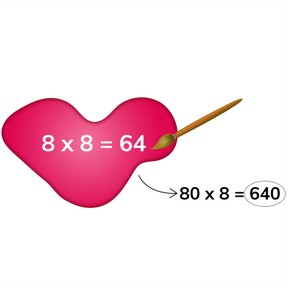Mental math to multiply numbers that are multiples of 10

# Mental math to multiply numbers that are multiples of 10

I can multiply numbers that are multiples of ten using mental math.8,000 schools use Gynzy92,000 teachers use Gynzy1,600,000 students use Gynzy

## General

In this lesson, students use mental math to quickly solve equations with multiples of ten like 40 x 80. First, they will solve the basic fact, then they will count the zeros and write them in after the answer to the basic fact.

## Standards

CCSS.MATH.CONTENT.3.NBT.A.3
CCSS.MATH.CONTENT.4.NBT.A.1

## Learning Objective

Students will be able to identify basic facts and use them to quickly multiply numbers that are multiples of 10.

## Introduction

The lesson begins with a review of mental math multiplication facts. Students will try to beat a timer to answer several basic facts before reviewing the multiples of ten.

## Instruction

Students learn how to multiply numbers that are multiples of ten by first finding and solving the basic fact and then counting and adding the zeros. Students will practice this skill by identifying basic facts, answering questions in two steps, and dragging the basic fact then answering equations. Students will also have the opportunity to practice with larger numbers as well as word problems.

## Quiz

Students respond to true/false and multiple-choice questions as well as a fill-in response.

## Closing

The lesson concludes with a true/false game in which students work in teams to decide if a question and answer is correct or incorrect. If they choose correctly, they score points for the team. Bonus points are earned for correcting an incorrect answer.

## Teaching tips

Students may have trouble with basic facts that have a zero in the answer, like 4x5=20. The lesson reminds them to write the answer to the basic fact first, then count the zeros.

### The online teaching platform for interactive whiteboards and displays in schools

• Save time building lessons

• Manage the classroom more efficiently

• Increase student engagement